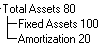## Aggregation (Consolidation Operator)

The Aggregation (Consolidation Operator) determines how child accounts aggregate to the parent account. Note that while this metadata aggregation property does not depend on, and is independent of the account type property, a relationship is required in order to apply the aggregation logic displayed in Table 10-8.

Note:

Financial Consolidation and Close has a consolidation script that performs the consolidation of one entity into another. Do not change the Consolidation Operator on Entities. If this Entity property is anything other than Ignore, the results will be incorrect.

The following table indicates how each Account Type behaves when totalled into a specific type of parent account within the balanced Balance Sheet hierarchy. For example, when aggregated, Asset account values are aggregated into parent Asset and Expense accounts, and subtracted from parent Liability and Revenue accounts.

Table 10-8 Required Aggregation (Consol Operator) between Child and Parent Accounts

Account Type Parent Account
Child Account Asset Liability Equity Revenue Expense

Asset

Addition

Subtraction

Subtraction

Subtraction

Addition

Liability

Subtraction

Addition

Addition

Addition

Subtraction

Equity

Subtraction

Addition

Addition

Addition

Subtraction

Revenue

Subtraction

Addition

Addition

Addition

Subtraction

Expense

Addition

Subtraction

Subtraction

Subtraction

Addition

Saved Assumption

Addition

Addition

Addition

Addition

Addition

Note that Saved Assumption accounts should not be used within the balanced Balance Sheet because they are non-financial accounts. Saved Assumption accounts can be added or subtracted when aggregating to their parent accounts as required.

This example illustrates how different account types are aggregated into parent accounts:In this example, Total Assets is an Asset account and the parent of Fixed Assets (an Asset account) and Amortization (a Liability account). When the accounts are aggregated into the parent account, the Fixed Assets value of 100 is added, the Amortization value of 20 is subtracted, and the resulting value for Total Assets is 80.

Changing the default Account Type and Consol Operator Settings

The default configuration of Financial Consolidation and Close assumes that for Assets and Expenses, a positive value represents a DR and a negative value represents a CR. For Revenue, Liabilities and Equity, a positive value represents a CR and a negative value represents a DR. This is the "normal sign" of the account. The system uses the combination of Account Type and Consol Operator in many of the system calculations to ensure correct aggregation for different client configuration requirements. The posting of journals (assigning DR and CR entries to a positive or negative value) also relies on the Account Type entry. Table 10-8 displays the default settings applied to seeded accounts.

In order to load data where a positive value is always a DR and a negative value is always a CR regardless of account (for example, a G/L extract), you will need to change both the Account Type and the Consol Operator. So change all "Revenue" accounts to Account Type "Expense" and change all "Liability" and "Equity" accounts to Account Type "Asset". Then change all Consol Operators to "Addition." For consistency, you should also change the parent accounts including the seeded members. Then run Validate Metadata again to verify that all settings are consistent and that system calculations will not be compromised.